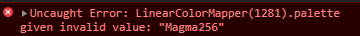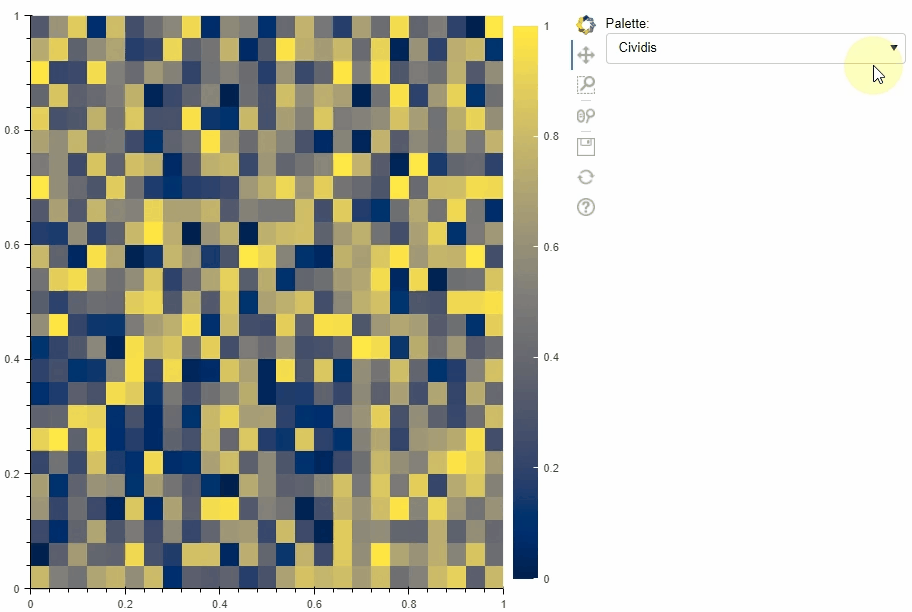# Choosing color_mapper using Select

I want to be able to change the palette using `select`.
Below is an example of my attempt. I just want to change the palette by taking the values out of the box.

``````import numpy as np
from bokeh.plotting import figure, show
from bokeh.models import Row
from bokeh.models import LinearColorMapper, BasicTicker, ColorBar, Select,CustomJS
from bokeh.models.widgets import RangeSlider
import bokeh
data = np.random.rand(10,10)
select = Select(title="Palette:", options=["Viridis256","Magma256"])
color_mapper = LinearColorMapper(palette=select.options, low=0, high=1)

figure = figure(x_range=(0,1), y_range=(0,1))
img = figure.image(image=[data], color_mapper=color_mapper,
dh=[1.0], dw=[1.0], x=, y=)

color_bar = ColorBar(color_mapper=color_mapper, ticker= BasicTicker(),
location=(0,0))

cmap_callback = CustomJS(args=dict(img=img), code='''
img.glyph.color_mapper.palette=this.value;
''')
select.js_on_change( 'value',cmap_callback)
show(Row(figure, select ))
``````

However, the selection doesn’t work. The console displays the following:I do not understand what the problem is, because I’m passing exactly what he considers invalid. Most likely the problem is in js. I have no proper experience with this language.

@KonradCurze The problem is that the ability to configure the palettes by string names is merely a convenience, and one that only exists on the Python side. The actual real type of the `palette` property is a list of colors. So if you are setting the property from JS you will need to assign values that are lists of colors that comprise the palette, instead of strings.

2 Likes``````import numpy as np
from bokeh.plotting import figure, show
from bokeh.models import Row
from bokeh.models import LinearColorMapper, BasicTicker, ColorBar, Select,CustomJS
from bokeh.io import output_notebook
import bokeh

output_notebook()

colormaps = ['Cividis', 'Magma', 'Inferno', 'Plasma', 'Viridis', 'RdBu', 'Spectral', 'RdYlBu']
bokeh_maps = {k: list(eval('bokeh.palettes.{}'.format(k)).values())[-1] for k in colormaps}###### This is where the main change is located

data = np.random.rand(25,25)

figure = figure(x_range=(0,1), y_range=(0,1))

color_mapper = bokeh.models.LinearColorMapper(palette=bokeh_maps[colormaps], low=0, high=1)
color_bar = bokeh.models.ColorBar(color_mapper=color_mapper, location=(0,0))

select = bokeh.models.Select(title='Palette:', options=colormaps, value=colormaps)

img = figure.image(image=[data], color_mapper=color_mapper,
dh=[1.0], dw=[1.0], x=, y=)

cmap_callback = CustomJS(args=dict(
bokeh_maps=bokeh_maps,
color_bar=color_bar,
),
code="""
color_bar.color_mapper.palette = bokeh_maps[cb_obj.value]
""")
select.js_on_change('value',cmap_callback)
show(Row(figure, select ))
``````
1 Like